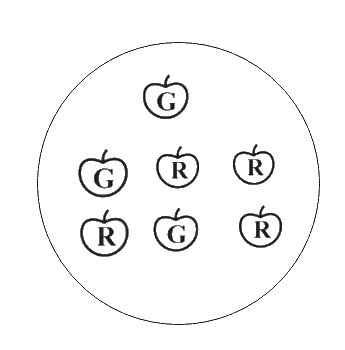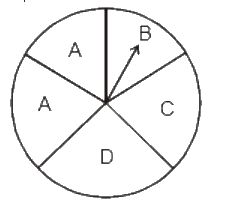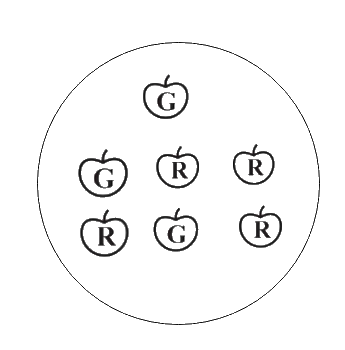In the verge of coronavirus pandemic, we are providing FREE access to our entire Online Curriculum to ensure Learning Doesn't STOP!

# Ex.5.3 Q3 Data Handling Solutions - NCERT Maths Class 8

Go back to  'Ex.5.3'

## Question

Find the:

(a) Probability of the pointer stopping on $$D$$ in (Question 1 (a))?

(b) Probability of getting an ace from a well shuffled deck of $$52$$ playing cards?

(c) Probability of getting a red apple? (See figure alongside)Video Solution
Data Handling
Ex 5.3 | Question 3

## Text Solution

What is known?

(i)  Spinning wheel image

(ii) Deck of $$52$$ playing cards

(iii) Image depicting Red and Green apples

What is unknown?

(i) Probability of the pointer stopping on $$D.$$

(ii) Probability of getting an ace from a well shuffled deck of $$52$$ playing cards.

(iii) Probability of getting a red apple

Reasoning:

Probability

\begin{align}= \! \frac{{{\text{Number of favourable outcomes}}}}{{{\text{Total number of outcomes}}}}\end{align}

Steps:

(a) In a spinning wheel, there are five pointers $$A, A, B, C, D.$$ So, there are five outcomes. Pointer stops at $$D$$ which is $$1$$ outcome. So, the probability of the pointer stopping on \begin{align}D = \frac{1}{5}\end{align}(b) There are $$4$$ aces in a deck of $$52$$ playing cards. So, there are $$4$$ events of getting an ace.

So, probability of getting an ace \begin{align} = \frac{4}{{52}} = \frac{1}{{13}}\end{align}

(c) Total number of apples $$= 7$$

Number of red apples $$= 4$$

So, probability of getting red apple \begin{align} = \frac{4}{7}\end{align}Learn from the best math teachers and top your exams

• Live one on one classroom and doubt clearing
• Practice worksheets in and after class for conceptual clarity
• Personalized curriculum to keep up with school